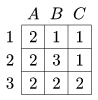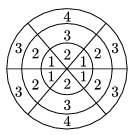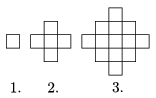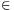Mathematical and Physical Journal
for High Schools
Issued by the MATFUND Foundation
 Already signed up? New to KöMaL?

# KöMaL Problems in Mathematics, February 2011

Show/hide problems of signs:## Problems with sign 'K'

Deadline expired on March 10, 2011.

K. 283. In a competition, participants form teams of six students. The composition of the teams is required to meet two conditions: 1. Every team should contain at least two girls and at least two boys. 2. Every team should contain at least two seventh-grade students and at least two eighth-grade students. A group of friends consists of three seventh-grade girls, four eighth-grade boys and two eighth-grade girls. In how many different ways may they enter a team in the competition?

(6 pont)

solution (in Hungarian), statistics

K. 284. An isosceles right-angled triangle of unit legs is cut into two pieces by a line perpendicular to the hypotenuse. One piece is a kite, the other piece is a triangle. What percentage is the area of the kite of the area of the original triangle?

(6 pont)

solution (in Hungarian), statistics

K. 285. The ratio of the six-digit numbersandis 55:54. Find the values of the digits a, b and c.

(6 pont)

solution (in Hungarian), statistics

K. 286. There is a dwarf standing on each field of a 3×3 board. 2 of the dwarfs are liars. The liars never tell the truth. The other 7 dwarfs always tell the truth. Each dwarf is asked how many of the ones standing on fields adjacent to his field tell the truth. (Adjacent fields have an edge in common.) The answers are shown in the table. (For simplicity, the rows and columns of the board are denoted by letters and numbers.) What may be the positions of the liars on the board?(6 pont)

solution (in Hungarian), statistics

K. 287. Ann's grandmother made a birthday cake for her grandchild. Before decorating it with almond paste figurines, she weighed the cake on her digital scales that display weights rounded to the nearest tenth of a kilogram. The reading was 3.4 kg. When she put as many identical almond paste figurines on the cake as the age of Ann in years, the reading changed to 3.6 kg. The true weight of each figurine is an integer multiple of 10 grams, but any one of them alone reads 0.1 kg on the scales. How old may Ann be and what may be the weight of the figurines?

(6 pont)

solution (in Hungarian), statistics

K. 288. We want to make a set of triangular dominoes. The stones are regular triangles with an integer from 0 to 5 in each vertex. If a stone has three different numbers on it, then they increase in clockwise order. How many domino stones will there be? (There may also be three identical numbers on a stone, or two identical and one different number.)

(6 pont)

solution (in Hungarian), statistics## Problems with sign 'C'

Deadline expired on March 10, 2011.

C. 1065. Solve the equation, wherefor a positive integer n.

(5 pont)

solution (in Hungarian), statistics

C. 1066. The measures of four interior angles of a pentagon are 120o. The lengths of the four consecutive sides opposite to these angles are 2, 8, 5, 5, in this order. How long is the fifth side?

(5 pont)

solution (in Hungarian), statistics

C. 1067. Solve the following simultaneous equations on the set of real numbers: |x-1|+|x+y|=6, |y-1|+|x+y+1|=4.

(5 pont)

solution (in Hungarian), statistics

C. 1068. A target consists of 18 fields bounded by three concentric circles and three line segments through the centre, as shown in the figure. The areas of the fields marked with the same number are equal, and the area of number 2 is half that of number 3. By what factor is number 4 greater in area than number 1?(5 pont)

solution (in Hungarian), statistics

C. 1069. The first three elements of a sequence of figures made up of squares are shown. Students were asked how many squares there were in the nth figure of the sequence, expressed in terms of n.a,

b) 1+(n-1).4,

c) 1+(1+2+...+(n-1)).4,

d) (n-1)2+n2.

(5 pont)

solution (in Hungarian), statistics## Problems with sign 'B'

Deadline expired on March 10, 2011.

B. 4332. If the numbering of the faces of an (ordinary cubical) die is correct, the sums of the numbers on the faces adjacent to any chosen face are equal. Is it possible to number the faces of a dodecahedral die 1 to 12 in the same way?

(3 pont)

solution (in Hungarian), statistics

B. 4333. The lengths of two sides of a triangle are 12 and 18. The lengths of two sides of another, similar but not congruent, triangle are also 12 and 18. Find the lengths of the third sides of the triangles.

(Suggested by Á. Besenyei, Budapest)

(3 pont)

solution (in Hungarian), statistics

B. 4334. Given a line and a point on it, let Z denote the set of those points of the line that are at integer distances from the given point. Prove that if H is any three-element subset of Z, then it is possible to partition Z into subsets congruent to H.

(Suggested by B. Bodor and A. Éles, Budapest)

(5 pont)

solution (in Hungarian), statistics

B. 4335. Two circles intersect at points B and C. A line touches each of them, the points of tangency are A1 and A2. Prove that.

(Suggested by M. Mester, Szeged)

(4 pont)

solution (in Hungarian), statistics

B. 4336. Regular triangles are drawn over the sides AB and BC of a parallelogram ABCD on the outside. Their third vertices are E and F, respectively. Show that the sum of the angles CED and AFD is 60o.

(Suggested by Sz. Miklós, Herceghalom)

(4 pont)

solution (in Hungarian), statistics

B. 4337. Find all real numbers p such that the equation x3-7x+p=0 has two real roots whose difference is 1.

(4 pont)

solution (in Hungarian), statistics

B. 4338. Four points A, B, C and D on a circle are selected at random, independently of each other. What is the probability that the chords AB and CD intersect?

(4 pont)

solution (in Hungarian), statistics

B. 4339. Is the inequality PA+PB+PC<DA+DB+DC true for every interior point P of a regular tetrahedron ABCD?

(4 pont)

solution (in Hungarian), statistics

B. 4340. Prove that the following inequality is true for all positive numbers a1,a2,...,an.(5 pont)

solution (in Hungarian), statistics

B. 4341. Find all pairs f(x), g(x) of polynomials of real coefficients such that f(x+1)g(x-1)-g(x+1)f(x-1)=1.

(Suggested by P. Kutas, Budapest)

(5 pont)

solution (in Hungarian), statistics## Problems with sign 'A'

Deadline expired on March 10, 2011.

A. 527. Find all real numbers p for which the equation x3+3px2+(4p-1)x+p=0 has two real roots with difference 1.

(5 pont)

solution (in Hungarian), statistics

A. 528. Let a, b and n be positive integers and let C be a finite set of integers. Suppose that every positive integer can be represented in the form axn+byn+c with some positive integers x, y and cC. Find the possible values of n.

(Proposed by Péter Kutas, Budapest)

(5 pont)

statistics

A. 529. There is given a circle k on the plane, a chord AB of k, furthermore four interior points, C, D, E and F, on the line segment AB. Draw an arbitrary chord X1X2 of k through point C, a chord Y1Y2 through D, a chord U1U2 through E, finally a chord V1V2 through F in such a way that X1, Y1, U1 and V1 lie on the same side of the line AB, andholds. Let Z be the intersection of the lines X1X2 and Y1Y2, and let W be the intersection of U1U2 and V1V2. Show that the lines ZW obtained in this way are concurrent or they are parallel to each other.

(5 pont)

statistics